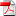# Branching Diffusion Representation of Semilinear PDEs and Monte Carlo Approximation

1
Mar

RR-FiME-16-03

### Pierre Henry-Labordère, Nadia Oudjane, Xiaolu Tan, Nizar Touzi, Xavier Warin

We provide a representation result of parabolic semi-linear PD-Es, with polynomial nonlinearity, by branching diffusion processes. We extend the classical representation for KPP equations, introduced by Skorokhod , Watanabe  and McKean , by allowing for polynomial nonlinearity in the pair (u;Du), where u is the solution of the PDE with space gradient Du. Similar to the previous literature, our result requires a non-explosion condition which restrict to \small maturity" or \small nonlinearity" of the PDE. Our main ingredient is the automatic di erentiation technique as in , based on the Malliavin integration by parts, which allows to account for the nonlinearities in the gradient. As a consequence, the particles of our branching diffusion are marked by the nature of the nonlinearity. This new representation has very important numerical implications as it is suitable for Monte Carlo simulation. Indeed, this provides the first numerical method for high dimensional nonlinear PDEs with error estimate induced by the dimension-free Central limit theorem. The complexity is also easily seen to be of the order of the squared dimension. The final section of this paper illustrates the efficiency of the algorithm by some high dimensional numerical experiments.

•rr-fime-16-03